SAT II Math I : Data Analysis and Statistics

Example Questions

Example Question #71 : Data Analysis And Statistics

A school district has determined that the most vaulable way to evaluate success is by using the median of all the test scores as the basis. With this in mind, find the median scores of 77, 80, 35, 76, 99, 95, 86, 65, 72, 56, and 21?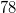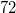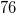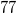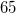Explanation:

The key to solving this problem is to first write the numbers in ascending order.

77, 80, 35, 76, 99, 95, 86, 65, 72, 56, 21

21, 35, 56, 65, 72, 76, 77, 80, 86, 95, 99

After this you will find the middle number of the set which turns out to be.

21, 35, 56, 65, 72, 76, 77, 80, 86, 95, 99

Example Question #72 : Data Analysis And Statistics

What is the median of the function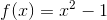when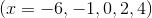?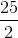Explanation:

We first plug each of thevalues into the function: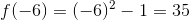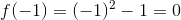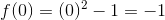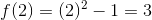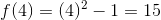Next, we put the answers in order from least to greatest: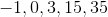Now, to find the median, we find the value that is in the middle of the data set. Since our data set has an odd number of entries the median will be the value that have an equal number of values to either side of it. In this particular case that value lies at the third entry which is 3.

Example Question #73 : Data Analysis And Statistics

Find the median of: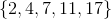Explanation:

Step 1: Define median...

The median of a set is the middle number, if the amount of numbers in a given set is odd. The median of a set with an even amount of numbers is the average of the two middle numbers in the set.

Step 2: Find the median of this set..

There is an odd number of numbers...

So, we take the middle number...

The middle number is.

So,is the median.

Example Question #74 : Data Analysis And Statistics

Find the median of the following set: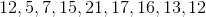Explanation:

To find the median, find the number that is in the middle of the set when all of the numbers are ordered from smallest to largest.First order the numbers from smallest to largest: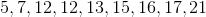In this case, the number in the middle of the set and our answer is:Example Question #75 : Data Analysis And Statistics

Find the median of the following set: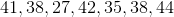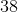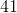Explanation:

To find the median, find the number that is in the middle of the set when all of the numbers are ordered from smallest to largest.First order the numbers in the set from smallest to largest: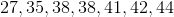In this case, our answer and the middle number of the set is:Example Question #76 : Data Analysis And Statistics

Find the median of the following set: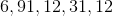Explanation:

To find the median, find the number that is in the middle of the set when all of the numbers are ordered from smallest to largest.First order the numbers in the set from smallest to largest: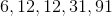In this case, our answer and the middle number of the set is:Example Question #77 : Data Analysis And Statistics

Identify the median of the following numbers: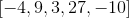Explanation:

Arrange the numbers in chronological order from least to greatest.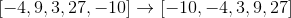The median is the central number.

The answer is:Example Question #78 : Data Analysis And Statistics

Identify the median: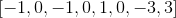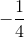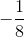Explanation:

Rearrange the data set from least to greatest.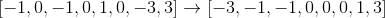Since this data set is even, the median the average of the central two numbers.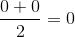The answer is:Example Question #1 : Mode

Find the mode of the following set of numbers: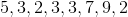Explanation:

The mode of a set of numbers is the one that appears most often. Thus, our answer is.

Example Question #2 : Mode

Find the mode of the following set of numbers: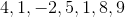The mode is the number that occurs the most frequently, so our answer is.